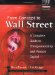# AppendixBasic Terms in Measurement

### Appendix Basic Terms in Measurement

The purpose of this section is to review and recall the basic terms in the measurement of returns and statistics which are used in valuations.

#### Basic Terms in Returns Measurement

• Net Present Value (NPV) NPV is the sum of all discounted flows (both positive and negative) from a project. NPV is used as a gauge for measuring the advisability of an investment; an investment is only worthwhile if NPV>0.

Example: We are contemplating investing \$100 in the present (C0) and expect the investment to yield (Cn) \$110 one year from now (n=1). We shall assume that the investment is "risky" and that the appropriate interest rate (k) is therefore 15%. Inserting these values in the formula reveals a negative NPV i.e., the investment is not worthwhile.

NPV = (-100) + 110/ (1 + 15%) = -4.348

• Rate of return The rate of return on an investment is the profit or loss made on the investment over the period of the investment, as a percentage of the initial amount invested. The rate of return is measured by dividing the sum of the cash flows (both positive and negative) by the amount of the investment. The rule is that an investment is worthwhile only when y>k, i.e., when the rate of return on the investment (y) is greater than the market return on such investment (k).

y = (C0 + Cn)/-C0

In the case of an initial investment (C0) of \$100 and a return (Cn) of \$110, the rate of return is:

y = (-100 + 110)/100 = 10%

If the appropriate interest rate (k) for an investment with such a risk profile is 15%, then the investment is not worthwhile.

• Internal Rate of Return (IRR) IRR is defined as the discount rate at which the NPV of the investment is zero.

IRR = (Cn/C0)1/n - 1

For example, in an investment of \$100 now which is expected to yield \$200 five years from now (n=5),

IRR = (200/100)1/5 - 1 = 14.9%From Concept to Wall Street: A Complete Guide to Entrepreneurship and Venture Capital
ISBN: 0130348031
EAN: 2147483647
Year: 2005
Pages: 131

Similar book on Amazon